# 总体概念

💭如果一个类中一个成员都没有，我们称之为空类。 那么，空类里真的什么都没有吗？

class Date{};//空类


⭕默认成员函数：用户没有显式实现，编译器会生成的成员函数称为默认成员函数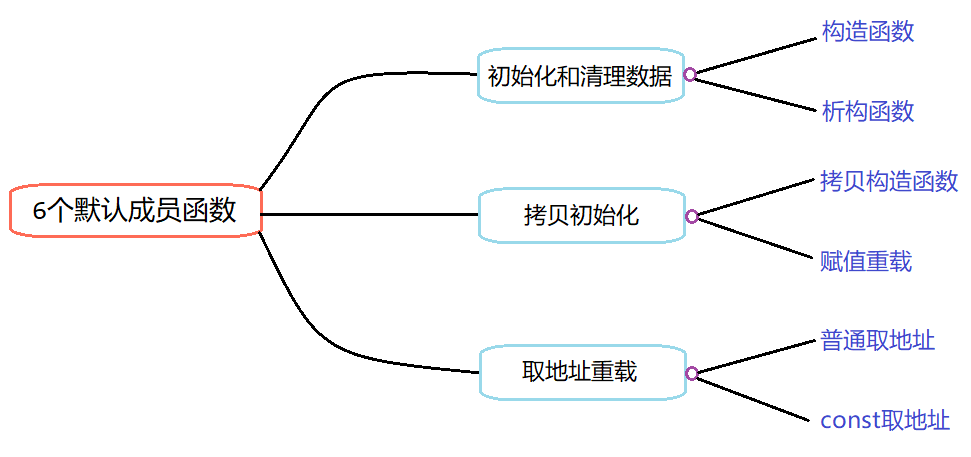# 1 构造函数

💭引入： 回想一下用C语言实现数据结构时，比如我们实现一个栈后，想要实例化一个栈的变量，那么定义完变量后还需要对其进行初始化（调用StackInit函数），这一步至关重要，但却稍显麻烦，甚至经常被我们所遗忘，这是个大问题。那么，有没有什么方法解决这个问题呢？
C++中的类定义了构造函数的概念，解决了这个问题。

## 概念

• 构造函数可以由程序员自己编写，也可以由编译器自动生成。名不副实，它的作用并不是开空间创建对象，而是初始化对象

• 构造函数是一个特殊的成员函数，名字与类名相同，创建类类型对象时由编译器自动调用，以保证每个数据成员都有一个合适的初始值，并且在对象整个生命周期内只调用一次。

## 特性

🎈构造函数有如下七个特性

1. 函数名与类名相同
2. 无返回值
3. 对象实例化时由编译器自动调用
4. 构造函数可以重载（关键用武之地在实现拷贝构造函数）
//4.
//以Date日期类的构造函数的实现作演示
class Date
{
public:
//无参构造函数
Date()
{}

//带参构造函数
Date(int year, int month, int day)
{
_year = year;
_month = month;
_day = day;
}

private:
int _year;

int _month;

int _day;
};

void TestDate()
{
//调用带参构造函数
Date d1(2022, 10, 12);

//调用无参构造函数
Date d2;

//注意：调用无参构造不能加空括号，否则就成了函数声明
//Date d3();//err
}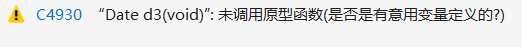带参的构造函数还可以与缺省值结合运用

//注意：二者不构成重载，不能同时存在
//半缺省构造函数
Date(int year, int month, int day = 1)
{
_year = year;
_month = month;
_day = day;
}

//全缺省构造函数
Date(int year = 2022, int month = 10, int day = 12)
{
_year = year;
_month = month;
_day = day;
}


1. 若在类中没有显式定义构造函数，C++编译器则会自动生成一个无参的构造函数，称为默认构造函数。一旦用户显示定义了，就不生成。
class Date
{
public:

//屏蔽显式定义的构造函数
//Date(int year, int month, int day)
//{
//	_year = year;
//	_month = month;
//	_day = day;
//}

private:
int _year;

int _month;

int _day;
};

void TestDate()
{
Date d;
}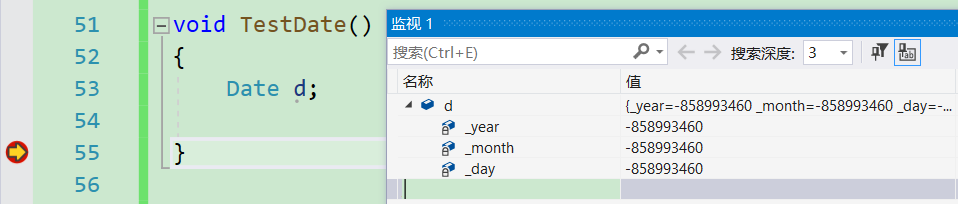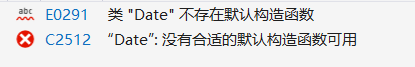1. 默认构造函数包括三种：
①不显式定义时，编译器自动生成的构造函数（自动生成的）
②无参构造函数（自己写的）
③ 全缺省构造函数（自己写的）
注意：默认构造函数只能有一个
class Date
{
public:
Date()
{}

Date(int year = 2022, int month = 10, int day = 12)
{
_year = year;
_month = month;
_day = day;
}

private:
int _year;

int _month;

int _day;
};

void TestDate()
{
Date d;
}

//编译失败，默认构造函数只能有一个，而这里有两个，一个是无参构造函数，另一个是全缺省构造函数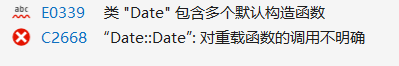1. 默认构造函数对内置类型成员的初始化是用随机值，对自定义类型成员的初始化是调用它的默认构造函数
（C++把类型分成内置类型(基本类型)自定义类型。内置类型就是语言提供的数据类型，如：int/char…，自定义类型就是我们使用class/struct/union等自己定义的类型）
//栈类
class Stack
{
public:
Stack(int n = 4)//默认构造函数
{
StDataType* tmp = (StDataType*)malloc(sizeof(StDataType) * n);
if (tmp == nullptr)
{
perror("malloc fail");
exit(-1);
}
//
_a = tmp;
_capacity = n;
_top = 0;
}

private:
int* _a;
int _top;
int _capacity;
};

//用两个栈模拟实现的队列类
class MyQueue
{
public:

//无显式定义的默认构造函数

private:
Stack _pushSt;

Stack _popSt;

int _size;
};

void TestMyQueue()
{
MyQueue q;
}


⭕通过Debug可以看到，两个Stack类的成员调用了它们自己的默认构造函数，而内置类型成员_size赋随机值0（因编译器而异，vs2019测试下赋的是0）

这种情况下必须保证自定义类型成员有默认构造函数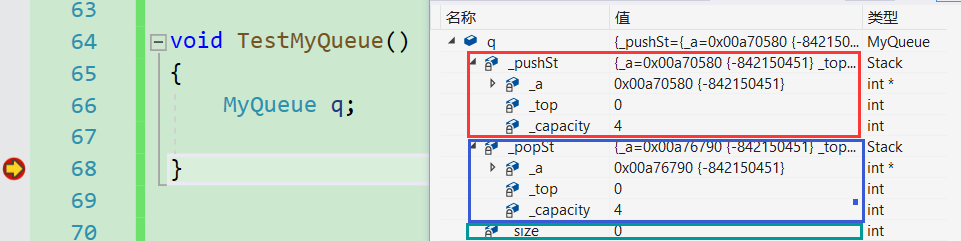💡C++11中，针对默认构造函数中内置类型初始化的不足之处（即用随机值初始化），打了一个补丁。即：内置类型成员变量在类中声明时可以给缺省值，如下：

class Date
{
public:

private:
// 都给缺省值
// 注意：这里不是初始化变量，只是一种声明
int _year = 2002;

int _month = 10;

int _day = 29;
};

void TestDate()
{
Date d;
}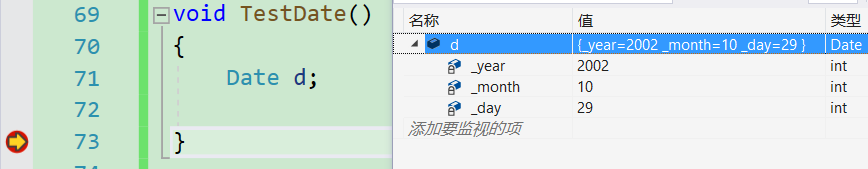## 初始化列表

💭引入： 实例化一个类对象时，会调用该类的构造函数对其进行初始化，给每一个成员变量一个适当的值。这和我们平时写代码初始化一个变量的行为类似。

class Date
{
public:

Date(int year, int month, int day)
{
_year = year;
_month = month;
_day = day;
}

private:
int _year;

int _month;

int _day;
};


⭕那么每一个成员变量是在哪进行初始化的呢？正如我们的标题 —— 初始化列表

1️⃣概念

📃初始化列表：

class Date
{
public:
Date(int year, int month, int day)
//初始化列表
: _year(year)
, _month(month)
, _day(day)
{}

private:
int _year;

int _month;

int _day ;
};


2️⃣特性

1. 一个成员变量在初始化列表中只能出现一次（即初始化只能一次）

2. 类中出现以下类型的成员变量时，必须在初始化列表中进行初始化
①const类型
②引用类型
③无默认构造函数的自定义类型

3. 所有成员变量都要走初始化列表进行初始化，就算不显式写初始化列表也会走，因此，为确保初始化的正确性，在实现类时尽量显式地写初始化列表。

💡总结

⭕注意：成员变量在类中声明次序就是其在初始化列表中的初始化顺序，与其在初始化列表中的先后次序无关

class A
{
public:
A(int a)
:_a1(a)
, _a2(_a1)
{}
void Print() {
cout << _a1 << " " << _a2 << endl;
}
private:
int _a2;
int _a1;
};
int main() {
A aa(1);
aa.Print();
}


⭕输出结果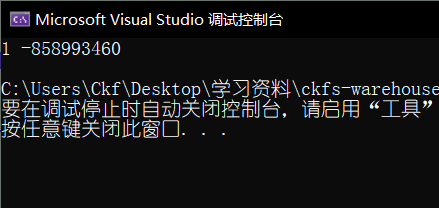# 2 析构函数

💭引入： 构造函数方便了我们创建和初始化对象，不用再像C语言一样需要自己调用初始化函数了。那么，知道了对象如何来的，对象又是如何消失的呢？在C语言中我们往往会调用一个Destroy函数来销毁对象，但它同样存在不少劣势。那么，在C++中，又引入了一个概念——析构函数，它与构造函数的作用是相反的。

## 概念

• 析构函数：析构函数并不是完成对对象本身的销毁（局部对象销毁工作是由编译器完成的），而是在对象销毁时会自动调用析构函数，完成对象中资源的清理工作。

## 特性

1. 析构函数名是在类名前加上字符 ~
2. 无参数无返回值类型
3. 一个类只能有一个析构函数。若未显式定义，系统会自动生成默认的析构函数。注意：析构
函数不能重载
4. 对象生命周期结束时，C++编译系统自动调用析构函数。
//用栈类作析构函数的演示
class Stack
{
public:
//Stack(int n = 4);

//栈的默认构造函数
~Stack()
{
cout << "~Stack()" << endl;//测试是否运行析构函数
free(_a);
_a = nullptr;
_top = 0;
_capacity = 0;
}

private:
int* _a;
int _top;
int _capacity;
};

void fun()
{
Stack s;
}

int main()
{
fun();
cout << "hello" << endl;
return 0;
}


⭕运行结果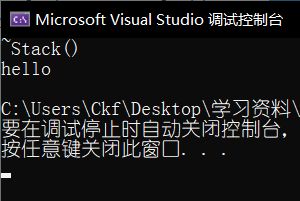1. 对于不显示实现，系统自动生成的默认析构函数，对于自定义类型成员调用它的析构函数。

💬如下代码

class Stack
{
public:
~Stack()
{
cout << "~Stack()" << endl;
free(_a);
_a = nullptr;
_top = 0;
_capacity = 0;
}
private:
int* _a;
int _top;
int _capacity;
};

class MyQueue
{
public:

private:
//内置类型
int _size;

//自定义类型
Stack _pushSt;
Stack _popSt;
};

void TestMyQueue()
{
MyQueue q;
}

int main()
{
TestMyQueue();

return 0;
}


⭕运行结果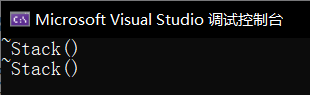💡总结

⭕注意：创建哪个类的对象则调用该类的析构函数，销毁哪个类的对象则调用该类的析构函数

1. 如果类中没有申请资源时（如成员变量都是内置类型），析构函数可以不写，直接使用编译器生成的默认析构函数，比如Date类；有资源申请时（如动态内存开辟），一定要写，否则会造成内存泄漏，比如Stack类。

💭构造函数与析构函数相辅相成，一个"生"对象一个"灭"对象，且由编译器自动调用，大大提高我们写代码时的简洁性、灵活性。

# 3 拷贝构造函数

💭引入： 若我们要定义一个变量与另一个变量相同，很简单，就像这样：int a =10;int b = a 即可。这是内置类型变量的方法。那么，如果这两个变量是自定义类型呢？也就是说，我们是否能用一个自定义类型变量去初始化另一个同类型的自定义类型变量呢？答案是肯定的。为了实现这种需求，C++引入了拷贝构造函数的概念。

## 概念

• 拷贝构造函数：是构造函数的重载函数，只有一个形参（还有一个隐式参数 this），该形参是对本类类型对象的引用（常用const修饰），在用已存在的同类类型的对象初始化新对象时由编译器自动调用。
class Date
{
public:
//Date类的拷贝构造函数
Date(const Date& d)
{
_year = d._year;
_month = d._month;
_day = d._day;
}

Date(int year, int month, int day)
: _year(year)
, _month(month)
, _day(day)
{
_year = year;
_month = month;
_day = day;
}

private:
int _year = 2002;

int _month = 10;

int _day = 29;
};

void testCopy()
{
Date d1(2022, 10, 16);

//两种调用拷贝构造的方式
Date d2(d1);
Date d3 = d1;
}

int main()
{
testCopy();
return 0;
}


📃通过Debug，观察到d1、d2、d3完全相同，说明d2、d3调用了拷贝构造函数。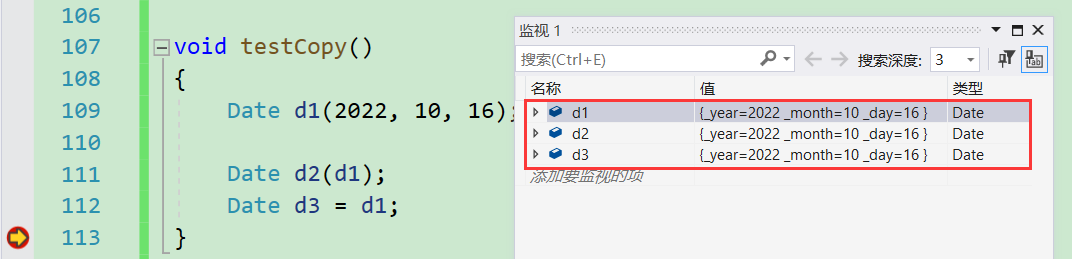## 特性

1. 拷贝构造函数是构造函数的重载函数
2. 拷贝构造函数的参数只有一个且必须是类类型对象的引用，使用传值方式编译器会直接报错，
因为会引发无穷递归调用
    //将拷贝构造函数改成传引用调用
Date(const Date d)
{
_year = d._year;
_month = d._month;
_day = d._day;
}


⭕引发无穷递归！！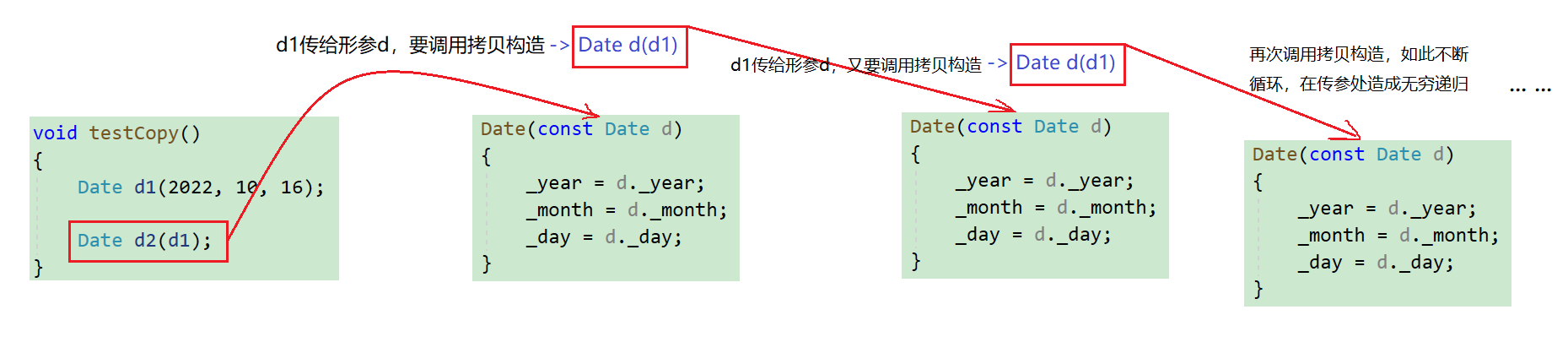1. 若未显式写拷贝构造函数，编译器会自动生成默认拷贝构造函数，默认的拷贝构造函数按对象内存存储字节序进行拷贝（类似memcpy），这种拷贝叫做浅拷贝，或者值拷贝。
class Date
{
public:
//屏蔽显式实现的拷贝构造函数
//Date(const Date& d)
//{
//	_year = d._year;
//	_month = d._month;
//	_day = d._day;
//}

Date(int year, int month, int day)
: _year(year)
, _month(month)
, _day(day)
{
_year = year;
_month = month;
_day = day;
}

private:
int _year = 2002;

int _month = 10;

int _day = 29;
};

void testCopy()
{
Date d1(2022, 10, 16);

Date d2(d1);
Date d3 = d1;
}

int main()
{
testCopy();
return 0;
}


⭕与显式写了拷贝构造的结果相同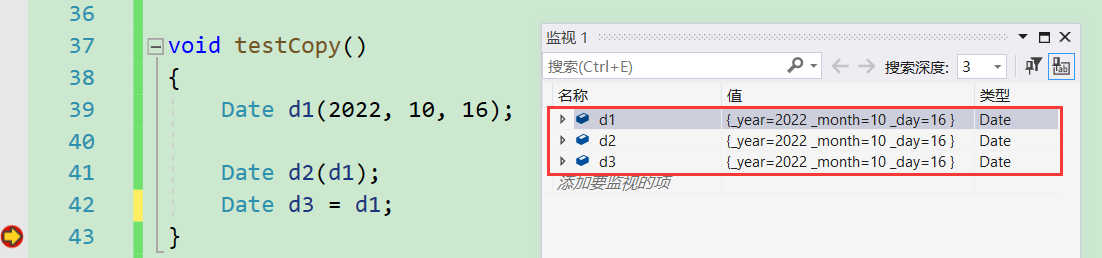1. 当然，编译器自动生成的默认拷贝构造函数并不能满足所有的类，大部分类的拷贝构造函数需要我们自己实现。

💬见下面的代码

//以Stack类为例，不显式地给Stack写拷贝构造函数
class Stack
{
public:
Stack(int n = 4)
{
StDataType* tmp = (StDataType*)malloc(sizeof(StDataType) * n);
if (tmp == nullptr)
{
perror("malloc fail");
exit(-1);
}
_a = tmp;
_capacity = n;
_top = 0;
}

~Stack()
{
cout << "~Stack()" << endl;
free(_a);
_a = nullptr;
_top = 0;
_capacity = 0;
}

private:
int* _a;
int _top;
int _capacity;
};

void testStack()
{
Stack s1(10);
Stack s2(s1);
}

int main()
{
testStack();
return 0;
}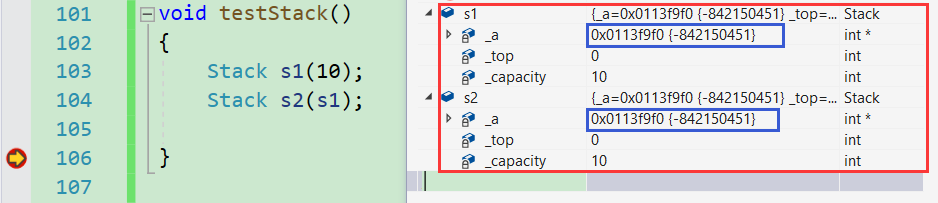⭕但是注意到，s1和s2中，成员_a的地址是一样的，也就是说，对s1和s2的操作会互相影响，而且最后调用析构函数时，会对同一块空间释放两次，导致程序崩溃！！

💡对于栈这种类的拷贝构造，我们希望的是两个类对象有两块互不干涉的独立空间，对一个对象的操作不会影响另外一个，那么就不能再使用默认拷贝构造的浅拷贝了，而应该自己实现一个深拷贝的拷贝构造函数。

💬实现方法如下：

class Stack
{
public:
Stack(int n = 4);

//栈的拷贝构造的实现
Stack(const Stack& s)
{
//申请空间
StDataType* tmp = (StDataType*)malloc(sizeof(StDataType) * s._capacity);
if (tmp == nullptr)
{
perror("malloc fail");
exit(-1);
}
_a = tmp;

//拷贝数据
memcpy(_a, s._a, sizeof(StDataType) * s._top);
_capacity = s._capacity;
_top = s._top;
}

~Stack()
{
cout << "~Stack()" << this << endl;
free(_a);
_a = nullptr;
_top = 0;
_capacity = 0;
}

private:
int* _a;
int _top;
int _capacity;
};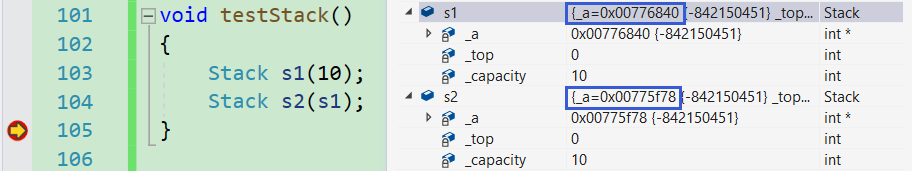💡总结

1. 拷贝构造函数典型调用场景：
① 使用已存在对象创建新对象
② 函数参数类型为类类型对象
③ 函数返回值类型为类类型对象
主要优化场景在2和3
class Date
{
public:

Date(int year, int month, int day)
: _year(year)
, _month(month)
, _day(day)
{
_year = year;
_month = month;
_day = day;
}

Date(const Date& d)
{
cout << "Date(const Date& d)" << endl;//测试调用了多少次拷贝构造
_year = d._year;
_month = d._month;
_day = d._day;
}

private:
int _year = 2002;

int _month = 10;

int _day = 29;
};

Date Test(Date d)
{
Date temp(d);//使用已存在对象创建新对象
return temp;//函数返回值类型为类类型对象
}

int main()
{
Date d1(2022, 10, 26);
Test(d1);//函数参数类型为类类型对象
return 0;
}

//一共调用了三次拷贝构造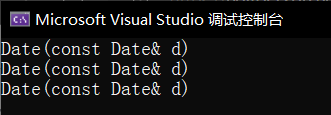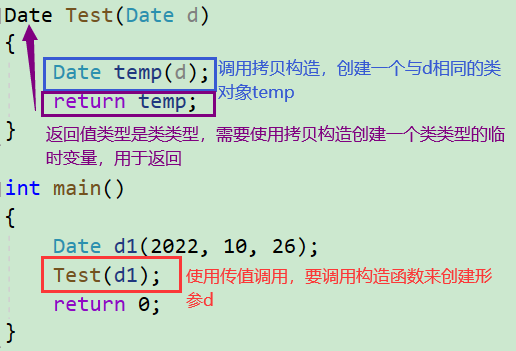# 4 赋值重载

## 4.1 运算符重载

💭引入： 平时我们若要判断两个数是否相等，可以直接使用'=='操作符。那么，若是两个同类型的类对象要判断是否相等该怎么办呢？当然可以写一个普通函数实现，但是，为了增强代码的可读性，C++引入了运算符重载的概念，以达到可以直接用常规的运算符（如：'==' '>' <' 等）来操作类对象。

• 运算符重载是具有特殊函数名的函数，也具有其返回值类型，函数名字以及参数列表，其返回值类型与参数列表与普通的函数类似。函数名字为：关键字operator后面接需要重载的运算符符号。

//若将运算符重载定义为全局函数，将无法访问类对象的private成员，不方便操作
//因此我们通常将运算符重载定义在类内，作成员函数

class Date
{
public:

Date(int year = 2022, int month = 10, int day = 16);

Date(const Date& d);

//Date类的 ‘==’ 重载
//注意：这里隐含的this指针（默认第一个形参）指向调用函数的类对象（‘==’的左操作数）。
bool operator==(const Date& d)
{
return _year == d._year
&& _month == d._month
&& _day == d._day;
}

private:
int _year;

int _month;

int _day;
};

void Test()
{
Date d1(2000,1,1);
Date d2(d1);

// d1 == d2  <==> d1.operator==(d2) 两种调用形式都可以

if (d1 == d2)
{
cout << "operator==" << endl;
}

if (d1.operator==(d2))
{
cout << "operator==" << endl;
}

}

int main()
{
Test();
return 0;
}


⭕运行结果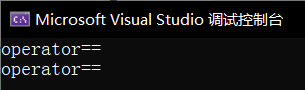## 4.2 赋值重载

1. 📃赋值运算符重载的格式（以Date类为例）：
• 参数类型：const Date& ➡ 传引用调用可以提高传参效率
• 返回值类型：Date& ➡返回引用可提高效率，有返回值是为了支持链式赋值
• 返回值为*this ➡ 符合链式赋值的含义
• 赋值前先检测是否为自己赋值自己，若是直接返回*this即可，避免多余操作。
class Date
{
public:

Date(int year = 2002, int month = 10, int day = 16);

Date(const Date& d);

bool operator==(const Date& d)
{
return _year == d._year
&& _month == d._month
&& _day == d._day;
}

//Date类的赋值重载
Date& operator=(const Date& d)
{
if (!(*this == d))
{
_year = d._year;
_month = d._month;
_day = d._day;
}
return *this;
}

//写一个打印日期函数，以便测试
void Print()
{
cout << _year << "年" << _month << "月" << _day << "日" << endl;
}

private:
int _year;

int _month;

int _day;
};

void Test()
{
Date d1(2000,1,1);
Date d2;
cout << "赋值前" << endl;
d1.Print();
d2.Print();
cout << endl;

cout << "赋值后" << endl;
d2 = d1;
d1.Print();
d2.Print();
cout << endl;

cout << "链式赋值" << endl;
Date d3(1999, 9, 9);
d1 = d2 = d3;
d1.Print();
d2.Print();
d3.Print();
cout << endl;
}

int main()
{
Test();
return 0;
}


⭕运行结果1. 用户没有显式实现时，编译器会生成一个默认赋值运算符重载，以值的方式逐字节拷贝。注意：内置类型成员变量是直接赋值的，而自定义类型成员变量需要调用对应类的赋值运算符重载完成赋值 （与自动生成的默认拷贝构造函数一样，是浅拷贝，因此我们需要根据需求判断是否需要自己显式实现）

2. 赋值运算符只能重载成类的成员函数不能重载成全局函数

class Date
{
public:
Date(int year = 1900, int month = 1, int day = 1)
{
_year = year;
_month = month;
_day = day;
}
int _year;
int _month;
int _day;
};

// 赋值运算符重载成全局函数，注意重载成全局函数时没有this指针了，需要给两个参数
Date& operator=(Date& left, const Date& right)
{
if (&left != &right)
{
left._year = right._year;
left._month = right._month;
left._day = right._day;
}
return left;
}
// 编译失败：
// “operator=”必须是非静态成员


💭拷贝构造函数和赋值重载功能类似，但也有本质上的区别。拷贝构造负责初始化（一个类对象只有一次），赋值重载则是负责将类对象赋值为一个同类型对象（一个类对象可有多次）。

# 📌const成员函数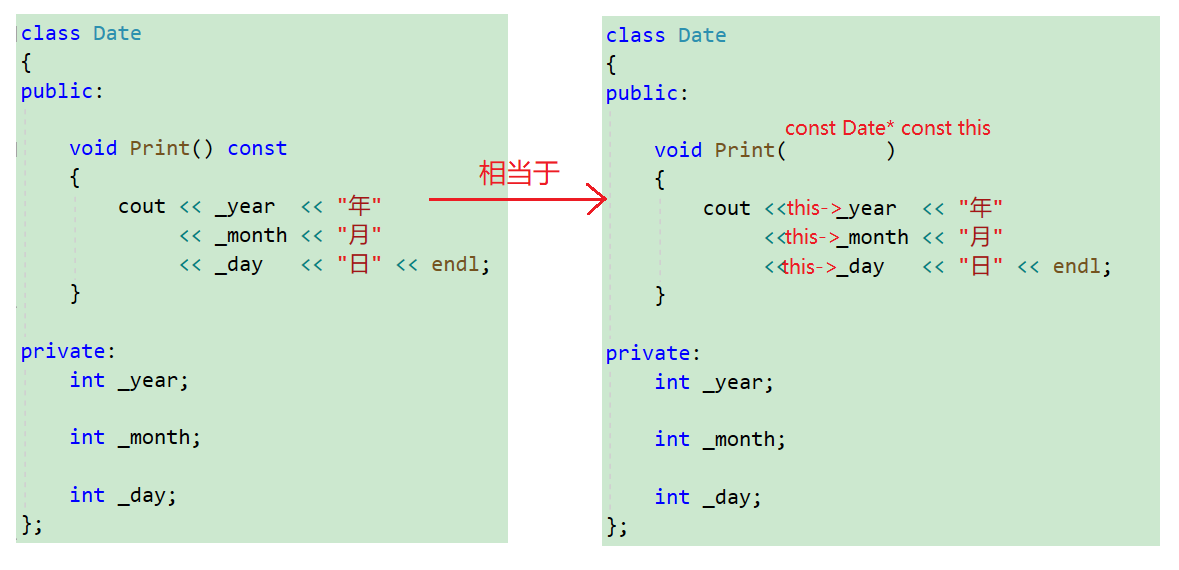💬看如下代码：

class Date
{
public:
Date(int year, int month, int day)
{
_year = year;
_month = month;
_day = day;
}
void Print()
{
cout << "Print()" << endl;
cout << "year:" << _year << endl;
cout << "month:" << _month << endl;
cout << "day:" << _day << endl << endl;
}
void Print() const
{
cout << "Print()const" << endl;
cout << "year:" << _year << endl;
cout << "month:" << _month << endl;
cout << "day:" << _day << endl << endl;
}
private:
int _year;
int _month;
int _day;
};
void Test()
{
Date d1(2022, 1, 13);
d1.Print();

const Date d2(2022, 1, 13);
d2.Print();
}

int main()
{
Test();
return 0;
}


⭕运行结果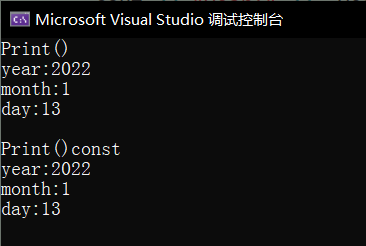const修饰成员改变了隐式形参this的类型，发生了函数重载，故传入const类型的this指针将会调用const修饰的成员函数，传入非const类型的this指针将会调用普通成员函数。这里是权限平移

class Date
{
public:
Date(int year, int month, int day)
{
_year = year;
_month = month;
_day = day;
}

//将无const修饰的成员函数去除

void Print() const
{
cout << "Print()const" << endl;
cout << "year:" << _year << endl;
cout << "month:" << _month << endl;
cout << "day:" << _day << endl << endl;
}
private:
int _year;
int _month;
int _day;
};
void Test()
{
Date d1(2022, 1, 13);
d1.Print();

const Date d2(2022, 1, 13);
d2.Print();
}

int main()
{
Test();
return 0;
}


⭕运行结果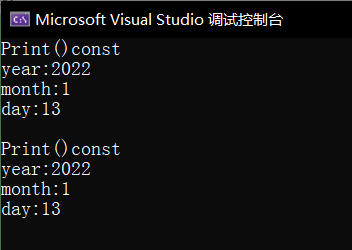d1、d2都调用了const成员函数Print，而d1传入的this指针并非const类型。说明权限可以缩小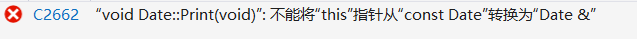💡总结

# 5 取地址重载和cons取地址重载

class Date
{
public:
Date* operator&()
{
return this;
}

const Date* operator&() const
{
return this;
}

private:
int _year;
int _month;
int _day;
};


# 6 日期类的实现（多种运算符重载）

// 获取某年某月的天数
int Date::GetMonthDay(int year, int month)
{
static int days = { 0,31,28,31,30,31,30,31,31,30,31,30,31 };
if (month == 2 && ((year % 4 == 0 && year % 100 != 0) || year % 400 == 0))
{
return 29;
}
else
{
return days[month];
}
}

// 赋值运算符重载
Date& Date::operator=(const Date& d)//权限缩小
{
if (*this != d)
{
_year = d._year;
_month = d._month;
_day = d._day;
}

return *this;
}

// 日期+=天数
Date& Date::operator+=(const int day)
{
if (day < 0)
{
return *this -= -day;
}
_day += day;
while (_day > GetMonthDay(_year, _month))
{
_day -= GetMonthDay(_year, _month);
_month++;
if (_month > 12)
{
_year++;
_month = 1;
}
}
return *this;
}

// 日期+天数
Date Date::operator+(const int day) const//注意这里的返回值是Date而不是Date&
{
Date d(*this);
d += day;
return d;
}

// 日期-=天数
Date& Date::operator-=(const int day)
{
if (day < 0)
{
return *this += -day;
}
_day -= day;
while (_day <= 0)
{
_month--;
if (_month < 1)//如果退位到年，月份需要先重置
{
_year--;
_month = 12;
}
_day += GetMonthDay(_year, _month);
}
return *this;
}

// 日期-天数
Date Date::operator-(const int day) const
{
Date d(*this);
d -= day;
return d;
}

//因为前置后置无法区分，C++规定后置的形参加一个int类型的参数

//后置比前置多了两次拷贝，效率较低

// 前置++
Date& Date::operator++()
{
*this += 1;
return *this;
}

// 后置++
Date Date::operator++(int)
{
Date tmp(*this);//第一次拷贝
*this += 1;
return tmp;//第二次拷贝
}

// 前置--
Date& Date::operator--()
{
*this -= 1;
return *this;
}

// 后置--
Date Date::operator--(int)
{
Date tmp(*this);
*this -= 1;
return tmp;
}

// >运算符重载
bool Date::operator>(const Date& d) const
{
if (_year > d._year)
{
return true;
}
else if (_year == d._year && _month > d._month)
{
return true;
}
else if (_year == d._year && _month == d._month && _day > d._day)
{
return true;
}
else
{
return false;
}
}

// ==运算符重载
bool Date::operator==(const Date& d) const
{
return  _year == d._year &&
_month == d._month &&
_day == d._day;
}

// >=运算符重载
bool Date::operator >= (const Date& d) const
{
return *this > d || *this == d;
}

// <运算符重载
bool Date::operator < (const Date& d) const
{
return !(*this >= d);
}

// <=运算符重载
bool Date::operator <= (const Date& d) const
{
return !(*this > d);
}

// !=运算符重载
bool Date::operator != (const Date& d) const
{
return !(*this == d);
}

int Date::operator-(const Date& d) const
{
//找出大天和小天
Date BiggerDay = *this;
Date SmallerDay = d;
int flag = 1;

if (BiggerDay < SmallerDay)
{
BiggerDay = d;
SmallerDay = *this;
int flag = -1;
}

//看小天自增多少次到大天，即为相距天数
int n = 0;
while (SmallerDay != BiggerDay)
{
++SmallerDay;
++n;
}
return n * flag;
}

//流提取和流插入的重载,使其适用于日期类对象

//操作数先后顺序不符
//解决方法：定义为全局函数

//链接错误的解决方法
//1.加static
//2.声明定义分离
//3.内联函数inline(最佳)

inline ostream& operator<<(ostream& out, const Date& d)
{
out << d._year << "年" << d._month << "月" << d._day << "日" ;

return out;
}

inline istream& operator>>(istream& in, Date& d)//d不能加const,因为要改变它的值
{
in >> d._year >> d._month >> d._day;

return in;
}

05-03646
02-26757
10-15984
08-17622
06-26748
08-12119
07-04199
10-22319
01-02116
09-18492
11-071087
05-02739
06-20739
07-29283
07-17116
04-25254
07-30185
08-11151

### “相关推荐”对你有帮助么？

•非常没帮助
•没帮助
•一般
•有帮助
•非常有帮助被折叠的  条评论 为什么被折叠?到【灌水乐园】发言¥2 ¥4 ¥6 ¥10 ¥20余额支付 (余额：-- )扫码支付获取中扫码支付点击重新获取扫码支付1.余额是钱包充值的虚拟货币，按照1:1的比例进行支付金额的抵扣。
2.余额无法直接购买下载，可以购买VIP、C币套餐、付费专栏及课程。余额充值